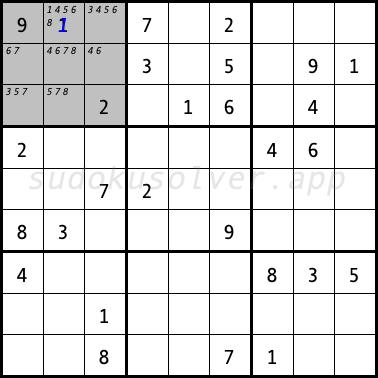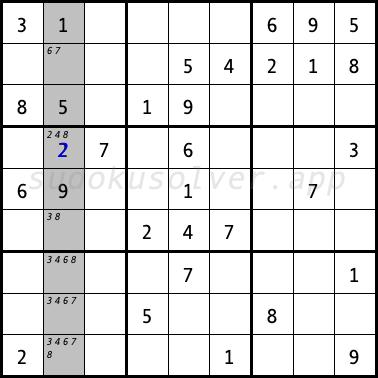# Hidden Single

A Hidden Single occurs when there is only one cell in a row, column or box in which a specific digit can placed. So this digit must be entered in this cell. This is the simplest and most commonly used technique, many diagrams can be solved using only this technique.

In the following sudoku diagram in the upper left box, the digit 1 can only be placed in the cell R1C2 (first row, second column, marked in blue) because from the remaining cells the digit 1 is eliminated by the digit 1 from the cells R2C9 (second row, ninth column), R3C5 (third row, fifth column) and R8C3 (eighth row, third column). So this is the only cell that this value can be placed in.In the next sudoku diagram in the second column the digit 2 can only be placed in the R4C2 cell (fourth row, second column) because in the other cells the digit 2 is eliminated by the cells R2C7 (second row, seventh column), R6C4 (sixth row, fourth column) and R9C1 (ninth row, first column).